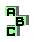POINT X(57)

 d De Longchamps ellipse r139 cevian quotient, Gergonne point, incenter r147 Nagel point, orthocenter, circumcenter, Mittenpunkt, De Longchamps point, point X(84) r156 Mannheim circles, incenter, circumcenter r168 excentral triangle, intouch triangle, homothetic triangles r279 Mittenpunkt, centroid, Gergonne point, complement r284 intouch triangle, orthic triangle, homothetic triangles r367 centroid, Mittenpunkt, isogonal points, desmic structure r668 intouch triangle, perspective,incircle, tangent circles r669 incircle, tangent circles, radical center r744 incenter, excenters, point X(173) r751 perspective, intouch triangle, Gergonne point r1138 circumcircle, incircle, circumcevian triangle, point X(55) r1594 incenter, point X(55), point X(165) r1805 incenter, circumcircle, Mannheim circles